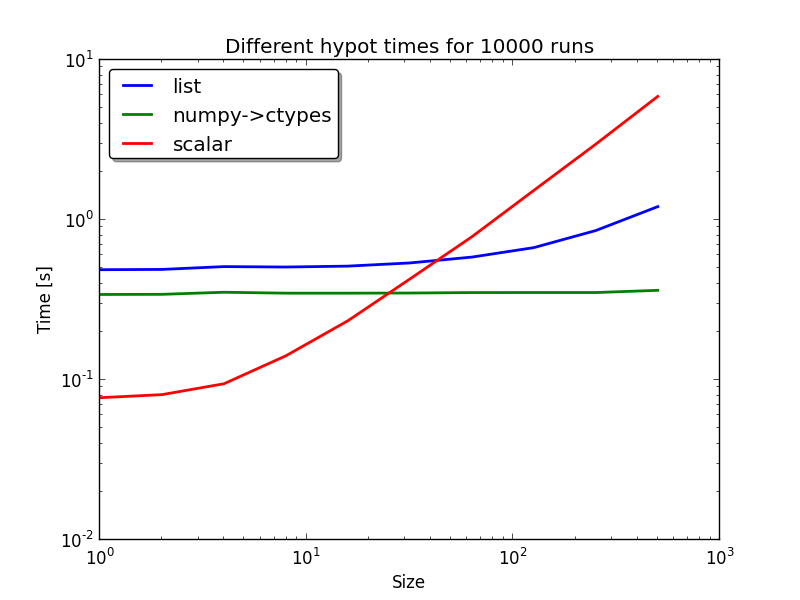# spacepy.toolbox.hypot¶

spacepy.toolbox.hypot(*args)[source]

compute the N-dimensional hypot of an iterable or many arguments

Parameters
argsmany numbers or array-like

array like or many inputs to compute from

Returns
outfloat

N-dimensional hypot of a number

Notes

This function has a complicated speed function.
• if a numpy array of floats is input this is passed off to C

• if iterables are passed in they are made into numpy arrays and comptaton is done local

• if many scalar agruments are passed in calculation is done in a loop

For max speed:
• <20 elements expand them into scalars
```>>> tb.hypot(*vals)
>>> tb.hypot(vals, vals...) #alternate
```
• >20 elements premake them into a numpy array of doubles

Examples

```>>> from spacepy import toolbox as tb
>>> print tb.hypot([3,4])
5.0
>>> print tb.hypot(3,4)
5.0
>>> # Benchmark ####
>>> from spacepy import toolbox as tb
>>> import numpy as np
>>> import timeit
>>> num_list = []
>>> num_np = []
>>> num_np_double = []
>>> num_scalar = []
>>> tot = 500
>>> for num in tb.logspace(1, tot, 10):
>>>     print num
>>>     num_list.append(timeit.timeit(stmt='tb.hypot(a)',
setup='from spacepy import toolbox as tb;
import numpy as np; a = *{0}'.format(int(num)), number=10000))
>>>     num_np.append(timeit.timeit(stmt='tb.hypot(a)',
setup='from spacepy import toolbox as tb;
import numpy as np; a = np.asarray(*{0})'.format(int(num)), number=10000))
>>>     num_scalar.append(timeit.timeit(stmt='tb.hypot(*a)',
setup='from spacepy import toolbox as tb;
import numpy as np; a = *{0}'.format(int(num)), number=10000))
>>> from pylab import *
>>> loglog(tb.logspace(1, tot, 10),  num_list, lw=2, label='list')
>>> loglog(tb.logspace(1, tot, 10),  num_np, lw=2, label='numpy->ctypes')
>>> loglog(tb.logspace(1, tot, 10),  num_scalar, lw=2, label='scalar')